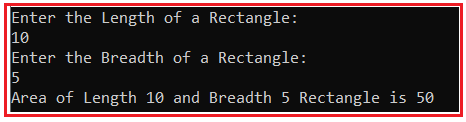# Area of Rectangle in C#

## Area of Rectangle Program in C# with Examples

In this article, I am going to discuss how to calculate the Area of a Rectangle in C# with Examples. So, here, first, we will discuss what is Rectangle, then we will discuss how to calculate the area of a rectangle and finally, we will see how to implement this program in C#.

##### What is a Rectangle?

A rectangle is a 2-Dimensional shape in geometry, having 4 sides and 4 corners. Its two sides meet at right angles. Thus, a rectangle has 4 angles, each interior angle is 90 ̊. The opposite sides of a rectangle have the same lengths and are parallel.

##### How to Calculate the Area of a Rectangle in C#?

Area of rectangle = length * breadth Or
A=L * B
Where A is the area of the rectangle
L is the length of the rectangle
B is the breadth of the rectangle

For Example: Let say: L i.e. Length =20 and B i.e. Breadth=5
Then the area of the Rectangle is 100
Explanation: Area of rectangle is the multiply of length (L) and breadth (B)
L=20, B = 5 So, L * B = 20 * 5 = 100

##### Logic to Calculate the Area of Rectangle in C#

For finding the area of the rectangle we simply need to multiply length and breadth. The following are the steps to follow to solve this problem.

1. First, we will define length L and breadth B.
2. Then we will define the area of rectangle A.
3. Then calculate the area of the rectangle by multiplying the length(L) and breadth(B).
4. After multiplying result will assign in A and show the output.
##### Example: Area of Rectangle Program in C#.

The following C# Program will calculate the area of the rectangle.

```using System;
public class AreaOfRectangle
{
public static void Main()
{
int Length = 15;
int Area = Length * Breadth;
}
}
```

OUTPUT: Area of Length 15 and Breadth 6 Rectangle is 90

##### Example: Area of Rectangle Program in C# by taking input from the user

The following C# Program will allow the user to input the length and breadth of the rectangle and then calculate the area and display it on the console.

```using System;
public class AreaOfRectangle
{
public static void Main()
{
Console.WriteLine("Enter the Length of a Rectangle: ");

Console.WriteLine("Enter the Breadth of a Rectangle: ");

int Area = Length * Breadth;